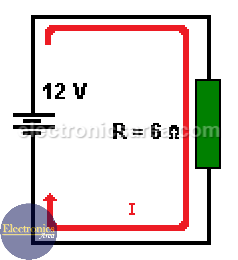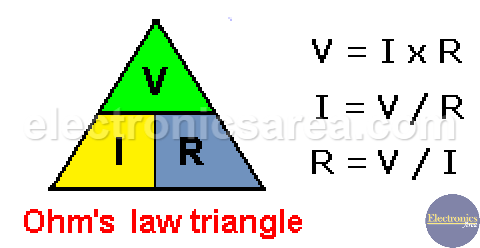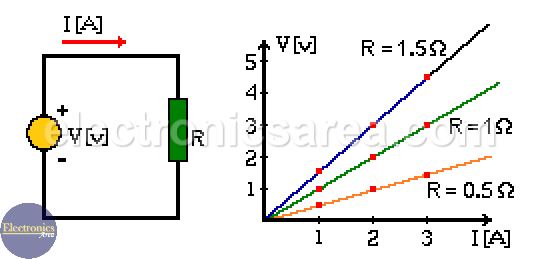# Ohm’s Law explanation

Ohm’s law is named after the German physicist Georg Ohm (1789-1854). Ohm’s law states that the voltage across a resistor is directly proportional to the current flowing through the resistance and directly proportional to the resistance of the circuit.

The Ohm’s Law formulas are: V = IR, I = V/R, and R= V/I.  It can be easily understood if we analyze a circuit where there is a battery (12 volts source of voltage) in series with a 6 ohms resistor.

## To find the Current: I = V/R

Therefore, the electric current flowing through the circuit (through the resistor) is: I = 12 Volts / 6 ohms = 2 Amperes.

## To find the Voltage: V = I x R

In the same way, we can solve the equation above for the voltage, and we get V = I x R (another expression for the Ohm’s law) so if we know the current and the resistor’s value, we can obtain the voltage between the terminals of the resistor, as follows: V = 2 Amperes x 6 ohms = 12 Volts.## To find the resistance R = V / I

As in the previous case, we can solve the equation for the resistance, and we get R = V / I. Then if we know the voltage in the resistor and the electric current passing through it, the resistor value is: R = 12 Volts / 2 Amps = 6 ohms

It is interesting to see that the relation between the electric current and the voltage in a resistor is always linear and the slope of this line is directly related to the value of the resistor. Thus, the bigger is the resistor value, the greater the slope will be.

The following triangle which is very similar to the formulas discussed above is used to remember the three expressions of the Ohm’s Law.There are 3 cases:

• If there is a fixed resistor value, an increment on the electric current means an increment on voltage.
• If there is a fixed voltage, an increment on the electric current is caused by a decrease in the resistance.
• If there is a fixed electric current, an increment on the resistance causes an increment on the voltage.

## Ohm’s Law for different resistor values

Looking at the picture we can see three lines with different slopes that correspond to three different resistors values.We can get, from these graphics, the current value for each resistor for a given voltage. We can also get the voltage value for each resistor for any current.

•
•
• 3
•
•
•
•
•
3
Shares
•
3
Shares
•
•
• 3
•
•
•
•